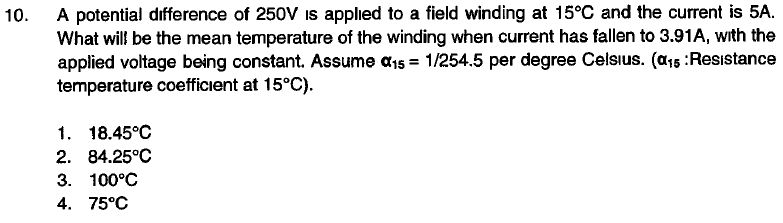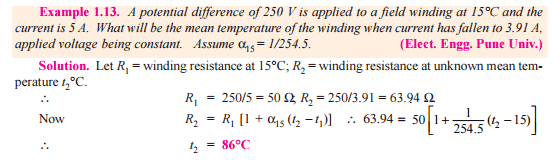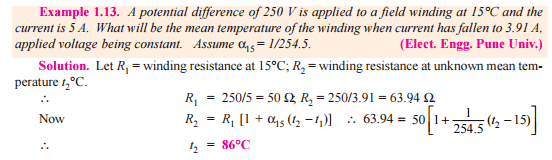# Mean temperature of winding when current falls

• DevonZA

## Homework Statement## Homework Equations

R2=R1(1+alpha(t2-t1))

## The Attempt at a Solution

R1=250/5=50ohms
R2=250/3.91=63.94ohms

R2=R1(1+alpha15degrees(t2-t1))
63.94=50(1+1/254.5(t2-15))
t2=

Now I found this online but the answers provided still don't match, 84.25 being the closest.When I manipulate the equation I get a totally different answer:
63.94=50(1+1/254.5(t2-15))
63.94/50=(1+1/254.5(t2-15))
1.2788/1+1/254.5=t2-15
1.274=t2-15
t2=1.274+15=16.274 degrees

where I am going wrong.
63.94/50=(1+1/254.5(t2-15))
1.2788/1+1/254.5=t2-15Is this from B.L. Theraja?

Not sure I found it online

Not sure I found it online
It is from B.L. Theraja. The page looks familiar. The red line in the above post is your error.

What have I done wrong though? Every way I enter it into my calculator yields an incorrect answer

63.94/50=(1+1/254.5(t2-15))
1.2788/1+1/254.5=t2-15[/\QUOTE]
This step is wrong. Check the sequence of operations.

Ah I got it :-) thank you